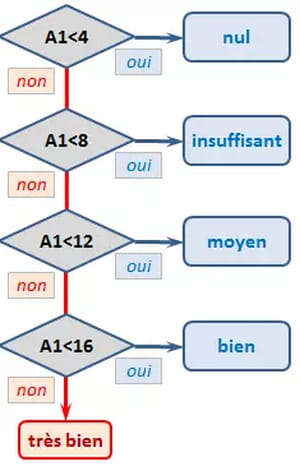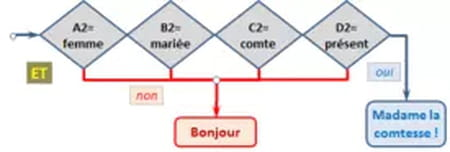# Excel IF, AND, OR, and NOT functionsThis article will explain how to use the conditional functions IF, AND, OR and NOT on Microsoft Excel. Each of these functions can be used as part of a formula in a cell to compare data samples in any number of columns or sheets in an Excel file. For ease of understanding, we have also included a visual map that illustrates how simple changes in each formula affects your results.

## IF function

The IF function allows you to apply a condition to your data whereby one result is returned if your condition is TRUE and another result if the condition is FALSE. IF functions can be either isolated, meaning that only one condition is applied to your data, or nested, where multiple criteria are applied to generate a TRUE or FALSE result.

### Isolated IF function

Here are two examples of an isolated IF function. In these examples, we are only applying one condition to generate our results:

If the grade in column A1 is greater than or equal to 19, then display 20; otherwise, display A1. = IF (A1> = 19, 20, A1)

If the data in A1 is "Discount", then subtract 10%, otherwise keep A1. = A1 * IF (A1 = "Discount"; 1-10%; 1)

### Nested IF functionHere are some examples of a nested IF function. In these cases, multiple IF functions are applied to the data, generating one of several responses depending on the data housed in the data constant. You'll notice that each criteria is separated by the use of a semicolon, and that the number of parentheses used at the end of our formula is dependent on the number of functions "nested" inside:

If the grade in A1 is greater than 12, then display "continue"; if A1 > 18, then display "very good", otherwise display "satisfactory". But if A1 <= 12, then display "improve".

=IF(A1>12;"continue," & IF(A1>18;"very good";"satisfactory");"improve")

or

=IF(A1<12;"continue";IF (A1<18;"continue, satisfactory";"continue, very good"))

If the grade in A1 is less than 4, then display "poor" ; if A1 is between 4 and 8, display "Unsatisfactory" ; if A1 is between 8 and 12, display "OK" ; if A1 is between 12 and 16, display "Good" ; if not, display "Very Good".

=IF(A1<4;"Poor";SI(A1<8;"Unsatisfactory";IF(A1<12;"OK";SI(A1<16;"Good";"Very Good"))))

## AND function

Unlike an IF function, which can function in isolation with one given condition, the AND function tests a plurality of user-defined conditions and returns TRUE if all data conditions are met, and FALSE if even one condition is not met.

Similar to other conditional formulas, you do have the capability to define your own results for TRUE and FALSE. Here's an example:

Show "The Countess!" if all of these conditions are met: A2 (sex) = woman, B2 (status) = married, C2 (spouse) = count and D2 (score) = present; if not, display "Hello!":

=IF(And(A2="woman";B2="married"; C2="count"; D2="present"); "The Countess!"; "Hello!")You'll note that this formula begins with the IF function. This use of IF signals to Excel that we are about to apply some sort of logical formula. Immediately following the IF function is our AND function and our data, grouped in the same set of parentheses to isolate the functions. Note that the "TRUE" condition ("The Countess!") is listed before the "FALSE" condition (Hello!). Both results are separated by a semicolon and are included in the parentheses.

## OR function

The OR function tests user-defined conditions and returns TRUE (or similar) if any of the conditions stipulated by the user are met, and FALSE (or similar) if none of the conditions apply. The OR function is much less absolute than the AND function. Here's an example:

Display "Pilot" if A3 contains one of the following conditions: plane, formula 1, motor; display "Conductor" if A3 contains car or work; if not, display "?"

=IF(OR(A3="plane";A3="formula 1"; A3="motor");"Pilot"; IF(OR(A3="car";A3="work");"Conductor";"?"))

## NOT function

The NOT function returns an opposite value of a user supplied logical value or expression.

The formula =IF(ENT(A3)=A3; "whole";"decimal") could also be written as =IF(NOT(ENT(A3)=A3); "decimal";"whole").

In the same way, the formula =IF(A4<>"French";"Foreign";"European") is equivalent to <bold>= IF(NOT(A4="French");"Foreign";"European").

## Combinations

The functions AND, OR, and NOT are most often associated with the IF function.

What's interesting (and satisfying) about these functions is that, together, they can be used to create a flow chart to serve as a visual representation of a problem's algorithm. When using these functions, ensure that all parentheses and semicolons are correctly placed to ensure the best results.